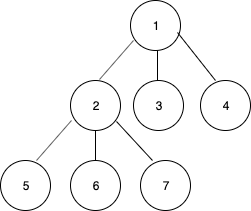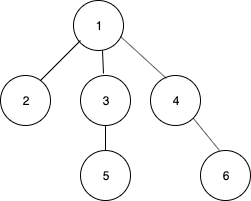Related Articles

# Inorder traversal of an N-ary Tree

• Difficulty Level : Medium
• Last Updated : 22 Jun, 2021

Given an N-ary tree containing, the task is to print the inorder traversal of the tree.

Examples:

Input: N = 3Output: 5 6 2 7 3 1 4
Input: N = 3Output: 2 3 5 1 4 6

Approach: The inorder traversal of an N-ary tree is defined as visiting all the children except the last then the root and finally the last child recursively.

• Recursively visit the first child.
• Recursively visit the second child.
• …..
• Recursively visit the second last child.
• Print the data in the node.
• Recursively visit the last child.
• Repeat the above steps till all the nodes are visited.

Below is the implementation of the above approach:

## C++

 `// C++ implementation of the approach``#include``using` `namespace` `std;` `// Class for the node of the tree``struct` `Node``{``    ``int` `data;` `    ``// List of children``    ``struct` `Node **children;``    ` `    ``int` `length;``    ` `    ``Node()``    ``{``        ``length = 0;``        ``data = 0;``    ``}` `    ``Node(``int` `n, ``int` `data_)``    ``{``        ``children = (Node**)``malloc``(``sizeof``(Node*)*n);``        ``length = n;``        ``data = data_;``    ``}``};` `// Function to print the inorder traversal``// of the n-ary tree``void` `inorder(Node *node)``{``    ``if` `(node == NULL)``        ``return``;` `    ``// Total children count``    ``int` `total = node->length;``    ` `    ``// All the children except the last``    ``for` `(``int` `i = 0; i < total - 1; i++)``        ``inorder(node->children[i]);` `    ``// Print the current node's data``    ``cout<< node->data << ``" "``;` `    ``// Last child``    ``inorder(node->children[total - 1]);``}` `// Driver code``int` `main()``{` `    ``/* Create the following tree``          ``1``        ``/ | \``        ``2 3 4``        ``/ | \``        ``5 6 7``    ``*/``    ``int` `n = 3;``    ``Node* root = ``new` `Node(n, 1);``    ``root->children = ``new` `Node(n, 2);``    ``root->children = ``new` `Node(n, 3);``    ``root->children = ``new` `Node(n, 4);``    ``root->children->children = ``new` `Node(n, 5);``    ``root->children->children = ``new` `Node(n, 6);``    ``root->children->children = ``new` `Node(n, 7);` `    ``inorder(root);``    ``return` `0;``}` `// This code is Contributed by Arnab Kundu`

## Java

 `// Java implementation of the approach``class` `GFG {` `    ``// Class for the node of the tree``    ``static` `class` `Node {``        ``int` `data;` `        ``// List of children``        ``Node children[];` `        ``Node(``int` `n, ``int` `data)``        ``{``            ``children = ``new` `Node[n];``            ``this``.data = data;``        ``}``    ``}` `    ``// Function to print the inorder traversal``    ``// of the n-ary tree``    ``static` `void` `inorder(Node node)``    ``{``        ``if` `(node == ``null``)``            ``return``;` `        ``// Total children count``        ``int` `total = node.children.length;``        ``// All the children except the last``        ``for` `(``int` `i = ``0``; i < total - ``1``; i++)``            ``inorder(node.children[i]);` `        ``// Print the current node's data``        ``System.out.print(``""` `+ node.data + ``" "``);` `        ``// Last child``        ``inorder(node.children[total - ``1``]);``    ``}` `    ``// Driver code``    ``public` `static` `void` `main(String[] args)``    ``{` `        ``/* Create the following tree``                   ``1``                ``/  |  \``               ``2   3   4``             ``/ | \``            ``5  6  7``        ``*/``        ``int` `n = ``3``;``        ``Node root = ``new` `Node(n, ``1``);``        ``root.children[``0``] = ``new` `Node(n, ``2``);``        ``root.children[``1``] = ``new` `Node(n, ``3``);``        ``root.children[``2``] = ``new` `Node(n, ``4``);``        ``root.children[``0``].children[``0``] = ``new` `Node(n, ``5``);``        ``root.children[``0``].children[``1``] = ``new` `Node(n, ``6``);``        ``root.children[``0``].children[``2``] = ``new` `Node(n, ``7``);` `        ``inorder(root);``    ``}``}`

## Python3

 `# Python3 implementation of the approach``class` `GFG:``    ` `    ``# Class for the node of the tree``    ``class` `Node:``        ``def` `__init__(``self``,n,data):``            ``# List of children``            ``self``.children ``=` `[``None``]``*``n``            ``self``.data ``=` `data``    ` `    ``# Function to print the inorder traversal``    ``# of the n-ary tree``    ``def` `inorder(``self``, node):``        ``if` `node ``=``=` `None``:``            ``return``        ` `        ``# Total children count``        ``total ``=` `len``(node.children)``        ` `        ``# All the children except the last``        ``for` `i ``in` `range``(total``-``1``):``            ``self``.inorder(node.children[i])``        ` `        ``# Print the current node's data``        ``print``(node.data,end``=``" "``)``        ` `        ``# Last child``        ``self``.inorder(node.children[total``-``1``])``    ` `    ``# Driver code``    ``def` `main(``self``):``        ``# Create the following tree ``        ``#          1``        ``#       /  |  \``        ``#      2   3   4``        ``#    / | \``        ``#   5  6  7``        ` `        ``n ``=` `3``        ``root ``=` `self``.Node(n, ``1``)``        ``root.children[``0``] ``=` `self``.Node(n, ``2``)``        ``root.children[``1``] ``=` `self``.Node(n, ``3``)``        ``root.children[``2``] ``=` `self``.Node(n, ``4``)``        ``root.children[``0``].children[``0``] ``=` `self``.Node(n, ``5``)``        ``root.children[``0``].children[``1``] ``=` `self``.Node(n, ``6``)``        ``root.children[``0``].children[``2``] ``=` `self``.Node(n, ``7``)``        ` `        ``self``.inorder(root)``        ` `ob ``=` `GFG() ``# Create class object``ob.main() ``# Call main function` `# This code is contributed by Shivam Singh`

## C#

 `// C# implementation of the approach``using` `System;` `class` `GFG``{` `    ``// Class for the node of the tree``    ``public` `class` `Node``    ``{``        ``public` `int` `data;` `        ``// List of children``        ``public` `Node []children;` `        ``public` `Node(``int` `n, ``int` `data)``        ``{``            ``children = ``new` `Node[n];``            ``this``.data = data;``        ``}``    ``}` `    ``// Function to print the inorder traversal``    ``// of the n-ary tree``    ``static` `void` `inorder(Node node)``    ``{``        ``if` `(node == ``null``)``            ``return``;` `        ``// Total children count``        ``int` `total = node.children.Length;``        ` `        ``// All the children except the last``        ``for` `(``int` `i = 0; i < total - 1; i++)``            ``inorder(node.children[i]);` `        ``// Print the current node's data``        ``Console.Write(``""` `+ node.data + ``" "``);` `        ``// Last child``        ``inorder(node.children[total - 1]);``    ``}` `    ``// Driver code``    ``public` `static` `void` `Main()``    ``{` `        ``/* Create the following tree``                ``1``                ``/ | \``            ``2 3 4``            ``/ | \``            ``5 6 7``        ``*/``        ``int` `n = 3;``        ``Node root = ``new` `Node(n, 1);``        ``root.children = ``new` `Node(n, 2);``        ``root.children = ``new` `Node(n, 3);``        ``root.children = ``new` `Node(n, 4);``        ``root.children.children = ``new` `Node(n, 5);``        ``root.children.children = ``new` `Node(n, 6);``        ``root.children.children = ``new` `Node(n, 7);` `        ``inorder(root);``    ``}``}` `// This code is contributed by AnkitRai01`

## Javascript

 ``
Output
`5 6 2 7 3 1 4 `

Attention reader! Don’t stop learning now. Get hold of all the important DSA concepts with the DSA Self Paced Course at a student-friendly price and become industry ready.  To complete your preparation from learning a language to DS Algo and many more,  please refer Complete Interview Preparation Course.

In case you wish to attend live classes with experts, please refer DSA Live Classes for Working Professionals and Competitive Programming Live for Students.

My Personal Notes arrow_drop_up